By McDonald, T.  |  Updated 24th of March 2020

Small errors in spreadsheets have been the cause of major problems in real life. Examining the kinds of common errors in spreadsheets helps us understand the general problems working with data can present. In order to understand these problems, it is a good idea to look at what a spreadsheet is and how equations can go wrong.

A spreadsheet consists of one or more sheets:
1. A sheet is a two-dimensional (array) of cells in which each cell is referenced by an index consisting of its column letter and row number.
2. A cell can contain data or a calculation (known as a formula).
3. There is a text box, known as a formula bar, in which the contents of a cell are displayed, and which is used for creating and editing the contents of the cell.

A sheet can contain one or more:
• Tables (sheets)
• A table is a two-dimensional array of data; some data is numeric, some is text.
• The table obviously has columns and rows.
• These need to be meaningful.
• Notes
• These are used much like comments in code and must be meaningful. For example, they could
• Other information.

Each of the values are in a separate cell. The value in a cell is called a datum. The value that you see is called a view because it may not be what is actually in the cell. For instance, the cell may contain a function, but the cell displays the result of the function and is what you view.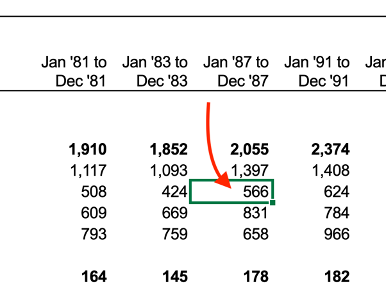Cell in spreadsheet.

Cells have an address so you can find them.  Down the left hand side are numbers and across the top are letters.  The number are the rows while the letters represent the columns. The cell address in this example is B8.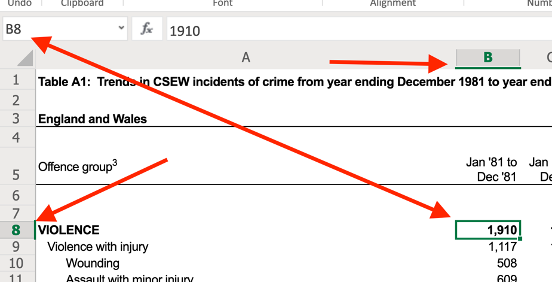Cell B8

## Cells can be auto updated by a formula

The equals sign indicates a formula is to follow. What follows the equals sign is called the formula.

Whenever you change a value of one or more of the cells mentioned in a calculation, the result will automatically change as a result. This ease of use is a potential weakness as you will see later.

Formulas can be copied to a range of other cells in the row starting from the selected cell by clicking on the handle in the bottom right corner, holding it and dragging it to the desired cell. Clicking on any of these cells will show the same formula.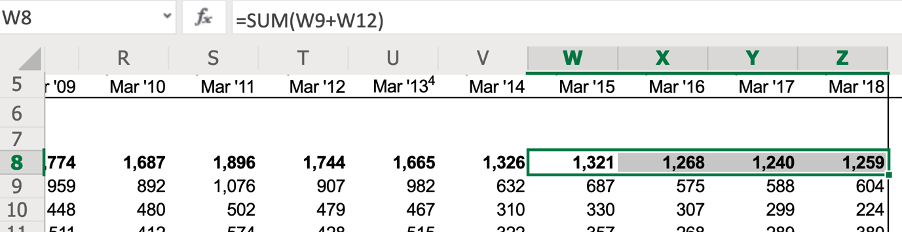A selected range of cells
You might also be starting to see how complicated a spreadsheet may become and how hard it might be to find errors in it.

An example of relative addressing is W8 or A10 (no \$ sign). The cell with the relative address is relative to another cell address. When a cell in a formula is copied, it will keep its relative address relative to the other address.

An example of absolute addressing is \$W\$8 or \$A\$10.

This will keep the cell address as W8 or A10 regardless of whether rows are deleted or added, which would change the address of the cell W8 to say W9 if a row before it was added.

W\$8, however, will allow the column to change to say F, but it will always refer to row 8.

## Formatting Cells

This allows the cells to take on the form of decimals, accounting, scientific etc.

## Built-in Statistical Functions

There are many of these. Here are some examples.
• =AVERAGE(B14:K14).
• Will calculate the average value from cell B14 to k14.
• =STDEVA(B14:K14).
• This is standard deviation.
• =COUNT(Cell:Cell)
• This will count ONLY cells with something in; blank cells don’t get counted.
• =COUNTIF(J3:J14, "Pass").
• This will count every cell if it contains ‘Pass’ from cells J3 to J14.
• This function is case sensitive.
• =AVERAGE(Cell: Cell) and =AVERAGEA(Cell: Cell)
• =AVERAGE calculates the numerical average value in a dataset, ignoring text. This function does not count the text values when dividing the added cells.
• =AVERAGEA calculates the numerical average value including text. This function does count the text values. It adds the numerical values together and then divides by the total number of cells including the text cells.

## Creating simple graphs

Selecting multiple cells: first click on the first cell and then hold shift and select the last cell you want in the range.
Next, click on insert and select a chart type. I have chosen 2D column chart (bar chart).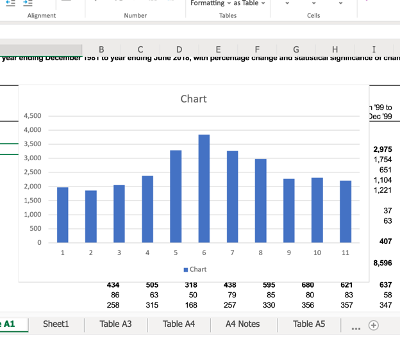Bar Chart

If you chose a line chart you will have to select chart and then select switch row/column.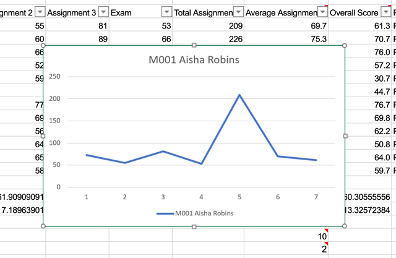Line Graph

## Excel can use IF statements

These are conditional statements with options that depend on the outcome of the condition.

=IF(I3>=50, "Pass", "Fail").

Spreadsheets do not scale very well. As they become larger and more complicated, they become prone to mistake that can break them.

• There are significant risks in using spreadsheets for solving real-world problems
• Financial disasters
• Technique disasters
• Complicated spreadsheets might be hard to find errors in it. The more complicated a spreadsheet becomes the more difficult it is to find errors.
• Formulas are not immediately obvious (magic numbers).
• Human error can break the spreadsheet; copying data is easy to do but is fraught with danger Copied data (whether within the same spreadsheet or in several spreadsheets). Not using relative addressing and absolute address in the correct way and for the correct reasons can lead to inaccurate calculations.
• Magic numbers. These are difficult to understand what they mean or stand for. For this reason, it is best to avoid them.
• Missing data
• Human error in general.
• Fraud – because of the mixture of code and data it is a great environment for fraud.
• Users can become overconfident because spreadsheet users do not look for errors. This includes being overconfident in the output of the spreadsheet.
• The underlining problem is that spreadsheet users have a good idea of the problem and motivation to solve it, but don’t run tests to check for validity because they see it as a waste of time.  Consequently, large amounts of spreadsheets have errors in them, which can then lead to errors in linked spreadsheets.  Data scientists and IT professionals know how to build robust systems that avoid these common problems.

## Now we know what the problems are what is the solution?

The follow can help but will not solve all problems:
1. Avoid copying data (use relative addressing, if possible).
2. Don't put 'magic numbers' in formulas.
3. Ensure that, if a value is likely to change, any other value that depends on that value should be calculated via a formula.
4. Document any cells that are used for holding variables occurring in formulas.
5. Use appropriate functions when there are missing data.
6. Avoid hiding data so that, if someone is new to the spreadsheet, they will be able to see all data.